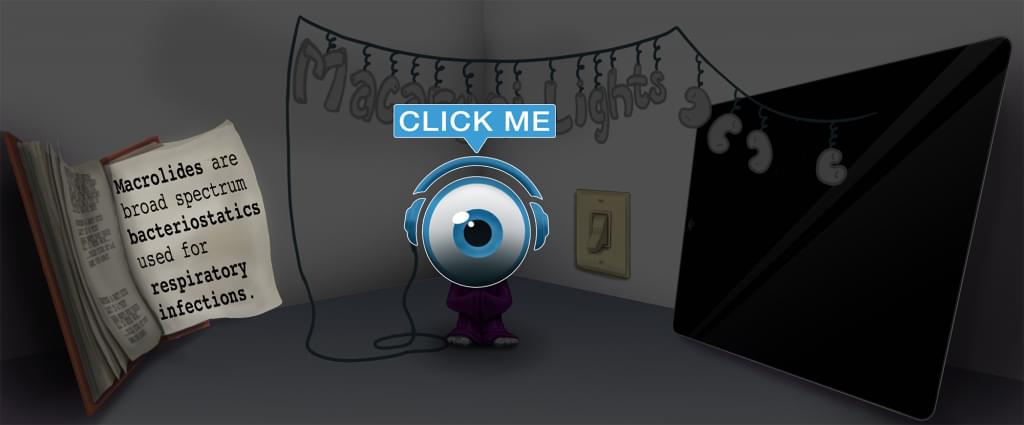# Master Relative Risk (RR) with Picmonic for Medicine

## With Picmonic, facts become pictures. We've taken what the science shows - image mnemonics work - but we've boosted the effectiveness by building and associating memorable characters, interesting audio stories, and built-in quizzing.

### Relative Risk (RR)

Relatives at Risk of DiseasePicmonic
Relative risk is a way to measure the effect of exposure to a risk factor on the probability of developing the outcome, or disease. It compares the outcome of an exposed group of individuals to a group of non-exposed individuals and quantifies how much more or less the exposed group is at risk for disease than the non-exposed group. Relative risk is utilized in cohort studies and is calculated by using values from a 2x2 contingency table in the formula RR = [a/(a+b)] / [c/(c+d)].
9 KEY FACTS
USE
Used in Cohort Studies
Cohosts

These observational studies identify two groups based on exposure; individuals exposed to a risk factor and individuals who lack exposure. The researcher can look retrospectively and see who developed the disease among the two groups, or the researcher can follow the two groups prospectively to track who will develop the disease. Cohort studies seek to measure the influence of risk factors on the development of disease and can quantify this influence with calculation of the relative risk.

FORMULA
Risk of Developing Disease
Risk of becoming Diseased-guy

This is another way of saying relative risk. If all else is held equal outside of exposure, relative risk quantifies how much more or less the exposed group is at risk for disease than the non-exposed group.

Equals =
Equals

Relative risk is equal to risk of developing disease in the exposed group divided by risk in the unexposed group.

Exposed Group

This group of subjects were all exposed to the studied risk factor

a / (a + b)
A-apple Divided by A-apple Plus B-bee body armor

The numerator of the formula is calculated by dividing subjects with exposure and disease (a) by the sum of the subjects with exposure and disease (a) and the subjects with exposure and no disease (b).

Divided by /
Divide

This division step should be carried out last. First, calculate the two terms found in the numerator and denominator respectively before dividing them.

Unexposed Group

This group of subjects were never exposed to the risk factor.

c / (c + d)
C-cat Divided by C-cat Plus D-dog

The denominator of the formula is calculated by dividing subjects who lack exposure but have disease (c) by the sum of the subjects who lack exposure but have disease (c) and the subjects who lack exposure and disease (d).

When Low Prevalence Odds Ratio Approximates Relative Risk
Down-arrow Diseased-girl throwing Odds-dice to Approximate Relatives Risk

If a disease has a low prevalence, i.e. rare diseases, the odds ratio approximates the relative risk. You can determine if prevalence is low if a lot of individuals have been exposed but only a few actually manifest with disease; in a 2x2 contingency table, there would be a very small “a” value with a very large “b” value, and thus a + b approximately equals b.

Unlock all 9 facts & definitions with Picmonic Free!

JOIN FREE

## Take the Relative Risk (RR) Quiz

Picmonic's rapid review multiple-choice quiz allows you to assess your knowledge.

START QUIZ NOW

## Our Story Mnemonics Increase Mastery and Retention

Memorize facts with phonetic mnemonics

Unforgettable characters with concise but impactful videos (2-4 min each)

### Ace Your Medical (MD/DO) Classes & Exams with Picmonic:

#### Over 970,000 students use Picmonic’s picture mnemonics to improve knowledge, retention, and exam performance.